Home > GC > Chapter 8 > Lesson 8.3.3 > Problem8-112

8-112.
1.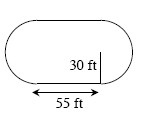The city of Denver wants you to help build a dog park. The design of the park is a rectangle with two semicircular ends. (Note: A semicircle is half a circle.) Homework Help ✎

1. The entire park needs to be covered with grass. If grass is sold by the square foot, how much grass should you order?

2. The park also needs a fence for its perimeter. A sturdy chain-linked fence costs about \$8 per foot. How much will a fence for the entire park cost?

3. The local design board has rejected the plan because it was too small. “Big dogs need lots of room to run,” the president of the board said. Therefore, you need to increase the size of the park with a zoom factor of 2. What is the area of the new design? What is the perimeter?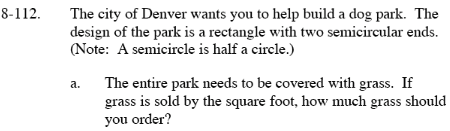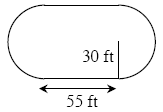Divide the park into three parts. Afterwards, find the area of each part then add them together.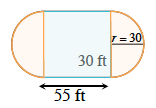Area of the rectangle = (l · w) = 55 · (2 · 30) = 3300 (where 2·30 is the diameter of the circle).

$\text{Area of one semicircle}=\frac{1}{2}\left ( \pi r^{2} \right )=450\pi$

Area of the dog park = semicircle + rectangle + semicircle = 450π + 3300 + 450π.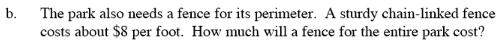Perimeter of the dog park = perimeter of semicircle + 55 + perimeter of semicircle + 55

$\text{Perimeter}=\frac{1}{2}2\pi r+55+\frac{1}{2}2\pi r+55$

After finding the perimeter, multiply your answer by 8 to determine the cost.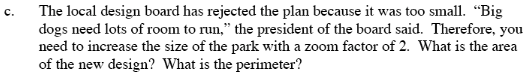The area of the new park = (the area of the old park)(the zoom factor)² ft
The perimeter of the new park = (the perimeter of the old park)(the zoom factor) ft